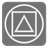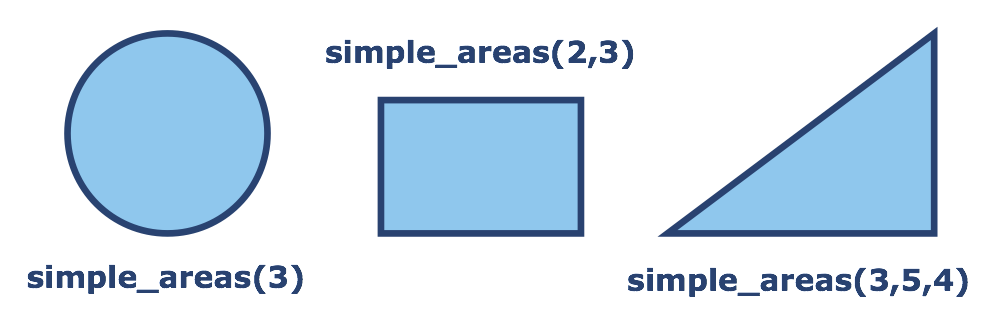# Simple Areas

Elementary+
English RU

Stephan works with simple forms when constructing something, and he need some programming tools to calculate his expenses. Let's take a trip back to our school days and pull out some simple geometry for this.

You should write a function to calculate the area of simple figures: circles, rectangles and triangles. You are give an arbitrary number of arguments and depending on this, the function calculates area for the different figures.

One argument -- The diameter of a circle and you need calculate the area of the circle.
Two arguments -- The side lengths of a rectangle and you need calculate the area of the rectangle.
Three arguments -- The lengths of each side on a triangle and you need calculate the area of the triangle.The result should be given with two digits precision as ±0.01.

Tips: Think about how to work with an arbitrary number of arguments .

Input: One, two or three arguments as floats or as integers.

Output: The area of the circle, the rectangle or the triangle as a float.

Example:

```simple_areas(3) == 7.07
simple_areas(2, 2) == 4
simple_areas(2, 3) == 6
simple_areas(3, 5, 4) == 6
simple_areas(1.5, 2.5, 2) == 1.5
```

How it is used: Python can be an useful tool for mathematics and science. You can easily implement any formulas or math algorithm with this simple and readable programming language.

Precondition:
0 < len(args) ≤ 3
all(0 < x ≤ 1000 for x in args)
For "triangle" cases the sum of the lengths of any two sides always exceeds the length of the third side.45
Settings
Code:
Other:
Invalid hot key. Each hot key should be unique and valid
Hot keys:
•  to Run Code: to Check Solution: to Stop:
CheckiO Extensions

CheckiO Extensions allow you to use local files to solve missions. More info in a blog post.

In order to install CheckiO client you'll need installed Python (version at least 3.8)

Install CheckiO Client first:

`pip3 install checkio_client`

`checkio --domain=py config --key=`

Sync solutions into your local folder

`checkio sync`

(in beta testing) Launch local server so your browser can use it and sync solution between local file end extension on the fly. (doesn't work for safari)

`checkio serv -d`

Alternatevly, you can install Chrome extension or FF addon

`checkio install-plugin`
`checkio install-plugin --ff`
`checkio install-plugin --chromium`

Read more here about other functionality that the checkio client provides. Feel free to submit an issue in case of any difficulties.